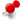# Mathematical equation as construction lineClassicListThreaded3 messagesOpen this post in threaded view
|

## Mathematical equation as construction line

 Is there any way to have LibreCad plot (or otherwise use) the "graph" of a mathematical equation as a construction (and/or design) line?  I wanted to use parametric equations for a cycloid as a construction line.